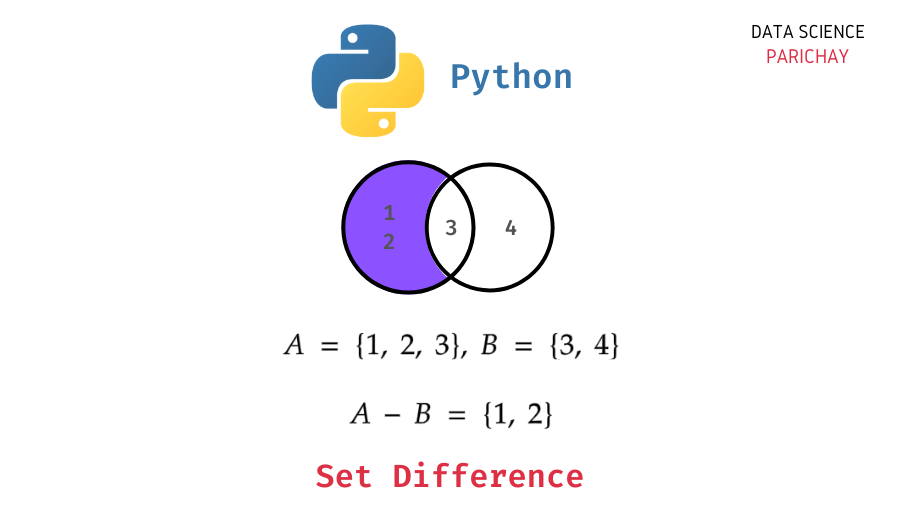# Set Difference in Python – With Examples

In this tutorial, we will look at how to compute the set difference in Python with the help of some examples.

The set difference operation between two sets, for example `A - B`, gives us the elements of set A which are not in set B. Let’s look at an example.

You can see that the resulting set from the `A - B` operation contains only the elements of set A which are not in set B.

Note that the set difference operation is not commutative. That is, `A - B` is not the same as `B - A` which results in a set containing the elements of B which are not in A. For example, computing `B - A` in the above example results in –

Thus, the set differences `A - B` and `B - A` are different from one another and may or may not be equal.

Python comes with a built-in set data structure to implement a set. It also has a number of additional functions to help you with common operations on sets such as union, intersection, difference, etc.

To get the difference between two sets in Python, you can use the set `difference()` function. The following is the syntax:

```# set difference operation a - b
a.difference(b)```

It returns a new set containing the elements of a which are not in b.

📚 Data Science Programs By Skill Level

Introductory

Intermediate ⭐⭐⭐

🔎 Find Data Science Programs 👨‍💻 111,889 already enrolled

Disclaimer: Data Science Parichay is reader supported. When you purchase a course through a link on this site, we may earn a small commission at no additional cost to you. Earned commissions help support this website and its team of writers.

```# create two sets
a = {1,2,3}
b = {3,7,2,6}
# set difference a - b
a.difference(b)```

Output:

`{1}`

We get the resulting set as {1} as 1 is the only element in set a which is not present in set b.

Alternatively, you can also use the subtraction operator `-` to compute set differences in Python. For example –

```# alternative method
print(a - b)```

Output:

`{1}`

We get the same result as above.

Note that the `-` operator for set difference only works on sets where as you can pass other iterables like lists, tuples, etc. to the set `difference()` function.

You can also get the difference between more than two sets. You can use the following syntax.

```# difference of more than two sets, for example, a,b,c,d
a.difference(b,c,d)```

Pass the sets (apart from the first one) as arguments to the `difference()` function. It returns a set containing the elements in set a which are not present in any of the passed sets. Let’s look at an example.

```# create four sets
a = {1,2,3,5,8}
b = {2,4,6}
c = {0,1,2,7}
d = {2,3,4}
# elements of set a not in b, c, and d
a.difference(b,c,d)```

Output:

`{5, 8}`

We get the resulting set as {5, 8} since 5 and 8 are the only elements in set a which are not present in the sets b, c, and d.

You can also use the `-` operator for set differences involving more than two sets.

```# alternative method
print(a-b-c-d)```

Output:

`{8, 5}`

We get the same result as above (note that sets are not ordered).

You might also be interested in –

•